##### Question

In: Statistics and Probability

# You roll a die 48 times with the following results. Number 1 2 3 4 5...

You roll a die 48 times with the following results.

 Number 1 2 3 4 5 6 Frequency 3 1 15 13 4 12

Use a significance level of 0.05 to test the claim that the die is fair.

## Solutions

##### Expert Solution

SOLUTION:

From given data,

Doing an observed/expected value table,

 O E (O-E)2 / E 3 8 (3-8)2 / 8 = 3.125 1 8 (1-8)2 / 8 = 6.125 15 8 (15-8)2 / 8 = 6.125 13 8 (13-8)2 / 8 = 3.125 4 8 (4-8)2 / 8 = 2 12 8 (12-8)2 / 8 = 2

Using ,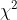=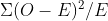= 3.125+6.125+6.125+3.125+2+2= 22.5

Degree of freedom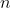= 6

df =-1 = 6-1 = 5

significance level =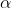= 0.05

Critical value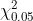=  11.07049769

Also, the p value is

p = 0.000421

Thus, comparingand[or, p and significance level], we REJECT THE NULL HYPOTHESIS.

There is significant evidence at 0.05 level that the die is not fair.

## Related Solutions

##### A die is rolled 60 times with the following results for the outcomes 1, 2, 3,...
A die is rolled 60 times with the following results for the outcomes 1, 2, 3, 4, 5, and 6, respectively: 13, 7, 6, 11, 10, and 13. Specify the null and alternative hypotheses. Use Minitab to perform the analysis. What is the conclusion? Show how to obtain by hand the found in the Minitab output. Demonstrate how to find the bounds on the p-value using a table
##### You roll a balanced die two times. Is the second roll independent of the first roll?
You roll a balanced die two times. Is the second roll independent of the first roll?
##### 1) Imagine you roll a fair 4 sided dice 4 times. what is the number of...
1) Imagine you roll a fair 4 sided dice 4 times. what is the number of possible outcomes as a sum of successes? 2) What is the probability you will roll 3 successes, if the result of a 4 is considered a success? 3) what is the probability you will roll 3 or 4 successes, of the results of a 4 is considered a success? 4) what is the probability you will roll 3 or 4 successes. of the result...
##### You roll a fair die three times. What is the probability of each of the​ following?...
You roll a fair die three times. What is the probability of each of the​ following? ​a) You roll all 4​'s. ​ b) You roll all even numbers.​ c) None of your rolls gets a number divisible by 3. ​ d) You roll at least one 2. ​e) The numbers you roll are not all 2​'s.
##### Suppose that you roll a die and your score is the number shown on the die....
Suppose that you roll a die and your score is the number shown on the die. On the other hand, suppose that your friend rolls five dice and his score is the number of 6’s shown out of five rollings. Compute the probability (a) that the two scores are equal. (b) that your friend’s score is strictly smaller than yours.
##### A die was rolled 300 times. The following frequencies were recorded. Outcome 1 2 3 4...
A die was rolled 300 times. The following frequencies were recorded. Outcome 1 2 3 4 5 6 Frequency 62 45 63 32 47 51 Do these data indicate that the die is unfair ? Use a 1% level of significance.
##### A die is rolled 50 times and the following are the outputs: 6 1 3 4...
A die is rolled 50 times and the following are the outputs: 6 1 3 4 2 6 3 5 1 3 6 1 6 6 3 3 6 5 2 4 1 6 5 3 1 2 5 2 1 2 4 1 4 1 5 5 6 6 2 1 1 2 5 6 5 5 6 3 1 3 What is the p-value of the chi-square test that the die is unbiased?
##### Assume we roll a fair four-sided die marked with 1, 2, 3 and 4. (a) Find...
Assume we roll a fair four-sided die marked with 1, 2, 3 and 4. (a) Find the probability that the outcome 1 is first observed after 5 rolls. (b) Find the expected number of rolls until outcomes 1 and 2 are both observed. (c) Find the expected number of rolls until the outcome 3 is observed three times. (d) Find the probability that the outcome 3 is observed exactly three times in 10 rolls given that it is first observed...
##### PROJECT C Roll a 6-sided die 800 times. If you do not desire to roll a...
PROJECT C Roll a 6-sided die 800 times. If you do not desire to roll a die manually, STATDISK can be used to simulate the process. To use STATDISK, go to “Data” at the top of the STATDISK window, and then choose “Dice Generator”. The “Dice Roll Random Sample Generator” window will appear. Then in that window, put 800 in for “Sample Size”, put 1 in for “Num Dice”, and put 6 in for “Num Sides”. Please disregard the “Random...